Algebra

# Inequalities - Solution Set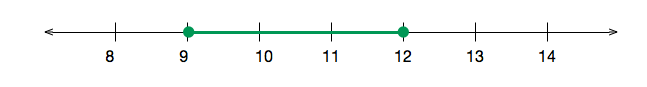Which inequality is represented in the above number line?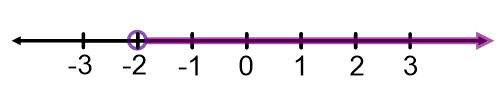Which inequality is represented in the above number line?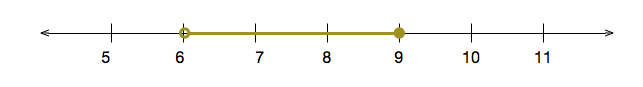Which inequality is represented in the above number line?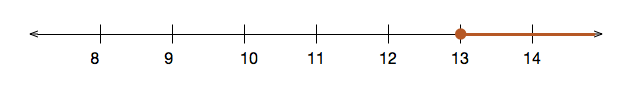Which inequality is represented in the above number line?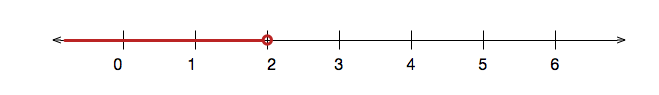Which inequality is represented in the above number line?

×